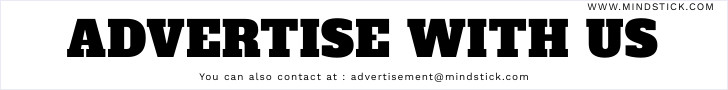# How to Create Windows Control Library and how to use in C#.Net

The Windows Control Library project template is used to create custom controls to use on Windows Forms .In the VS .NET IDE environment, controls can be found by clicking the toolbox icon. Windows controls can be either UI controls like "Edit" , "Label" , "ListBox" ,”TextBox”,”Button”etc (Found under the Windows Forms Tab ) .To create a control under the VS .NET IDE environment.
 With the help of Windows Form Control Library user can create own control. In this demonstration creating user control that will perform mathematical operation on two numbers.

##### Step:
•   Select Window from Installed Templates in Visual C#
•   Select Window From Control Library
•   Click OK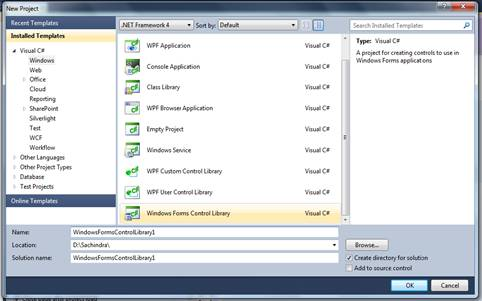After click OK button new Window will appear, on user control  simply drag and drop four button on it and button will be “Add”,”Subtract”,”Multiply” and ”Division” as  shown below: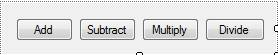##### Code:
`public partial class UserControl1 :  UserControl    {         publicUserControl1()         {            InitializeComponent();         }         intnum1=0, num2=0, res=0;//declaring three member variables of int type         Control ctrl1, ctrl2, ctrl3;//declaring three member variables of Control         stringname1, name2;//declaring three member variables of string that will store textBox name         private void UserControl1_Load(objectsender, EventArgse)         {         }         public stringTextBoxName1//creating property for textBox         {             get            {                 return name1;//this line will return textBox name            }             set            {                name1=value;//this line will set textBox name            }         }         public stringTextBoxName2//creating property for textBox         {             get            {                 return name2;//this line will return textBox name            }             set            {                name2=value;//this line will set textBox name            }         }         private voidbtnAdd_Click(objectsender,  EventArgse)         {              ctrl1= (TextBox)FindForm().Controls[name1];//in this line FindForm() method will find textBox control from the Form where the name of textBox is  store in name1            ctrl2= (TextBox)FindForm().Controls[name2];//in this line FindForm() method will find textBox control from the Form where the name of textBox is  store in name2             if (ctrl1==null|| ctrl2==null)//checking condition             {                 MessageBox.Show("TextBox can not blank");//it will show message                 return;//return the execution            }             try//            {                MyControl();//this a function                 res =num1+num2;//adding variable value and storing in res                Display();//this a function               }             catch (Exception ex)            {                 MessageBox.Show(ex.Message);//it will show message if exeception occurs            }              }         private voidbtnSub_Click(objectsender,  EventArgse)         {            ctrl1= (TextBox)FindForm().Controls[name1];            ctrl2= (TextBox)FindForm().Controls[name2];             if (ctrl1==null|| ctrl2==null)            {                 MessageBox.Show("TextBox can not blank");                 return;            }             try            {                MyControl();                res =num1-num2;//storing value in res after subtraction                Display();              }             catch (Exception ex)            {                 MessageBox.Show(ex.Message);            }           }                private voidbtnDiv_Click(objectsender,  EventArgse)         {            ctrl1= (TextBox)FindForm().Controls[name1];            ctrl2= (TextBox)FindForm().Controls[name2];                        if (ctrl1==null|| ctrl2==null)            {                 MessageBox.Show("TextBox can not blank");                 return;            }             try            {                MyControl();                res =num1/num2;//storing value in res after division                Display();                          }             catch (Exception ex)            {                 MessageBox.Show(ex.Message);            }         }          private void UserControl1_Load_1(objectsender, EventArgse)         {                     }               public voidMyControl()         {            ctrl3= (TextBox)FindForm().Controls["textBox3"];//in this line FindForm() method will find textBox control from the Form where the name of textBox is textBox3            num1=Convert.ToInt32(ctrl1.Text);//Converting in integer and storing in num1            num2=Convert.ToInt32(ctrl2.Text);//Converting in integer and storing in num2         }         public voidDisplay()         {            ctrl3.Text= Convert.ToString(res);//in this line res is storing as a text in textBox3         }           private voidbtnMul_Click_1(objectsender,  EventArgse)         {            ctrl1= (TextBox)FindForm().Controls[name1];            ctrl2= (TextBox)FindForm().Controls[name2];               if (ctrl1==null|| ctrl2==null)            {                 MessageBox.Show("TextBox can not blank");                 return;            }             try            {                MyControl();                res =num1*num2;//storing value in res after multiplication                Display();              }             catch (Exception ex)            {                 MessageBox.Show(ex.Message);            }         }    }  `
##### Step:
•    Right  Click on the Toolbox
•    Select Choose Items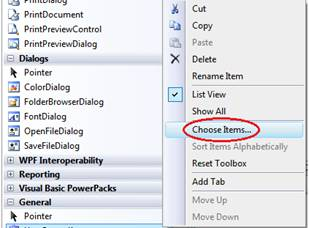After the “Choose Items” new toolbox “Choose Toolbox items” will be open click on the browse button and you have to specify where user control folder is.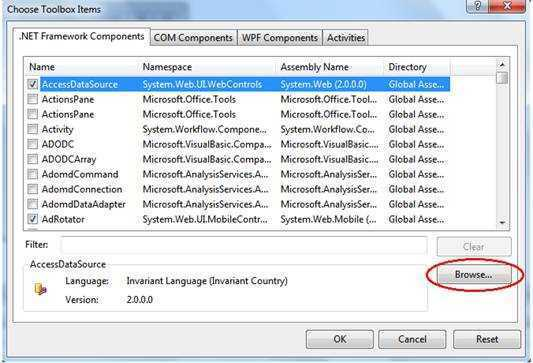##### After Select user control folder follow this step:
•   Open folder
•   Open sub folder (bin)
•   Open bin sub folder (Debug)
•   Open  Debug folder
•   In Debug folder open  user control ( .dll)
•   Then click on OK

After that we can see user control(UserControl1) has added in ToolBox, when we want to use this user control simply drag and drop on the form as shown below: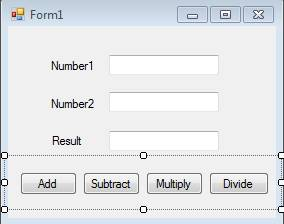This demonstration control can perform mathematical operation (Add, Subtract, Multiply and Divide) and result will be show in result TextBox as shown below.##### Code
`   public partial class Form1 :  Form    {         publicForm1()         {            InitializeComponent();         }         private void textBox1_TextChanged(objectsender, EventArgse)         {            userControl11.TextBoxName1=txtName1.Name;//this line will take textbox name and store in user control property TextBoxName1         }         private void textBox2_TextChanged(objectsender, EventArgse)         {            userControl11.TextBoxName2=txtName2.Name;//this line will take textbox name and store in user control property TextBoxName2         }         private voidForm1_Load(objectsender,  EventArgse)         {           }         private void textBox3_TextChanged(objectsender, EventArgse)         {           }                 private void userControl11_Load_3(objectsender, EventArgse)         {           }    }`

Last updated:5/19/2020 1:27:25 AM#### Sachindra Singh